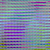# Cant plot image of tensors

i am converting numpy matrix to tensors and plotting them to google colab.as a numpy array it works fine but as a tensor it does not:
i have a array of tensors x:

``````X = torch.Tensor([i for i in train_data]).view(-1, 3, 50, 50)
``````

then i am plotting firs element of this array like this:

``````c2_imshow(X.numpy().transpose(1, 2, 0))
``````

but instead of dog picture it outputs something else like this:how can i output real picture(relevant)

i am converting numpy matrix to tensors and plotting them to google colab.as a numpy array it works fine but as a tensor it does not:

So:
numpy->tensor->numpy works but
tensor->numpy doesn’t?
Are you sure you are applying a proper reshaping?
c2_imshow is cv2? are you aware it’s bgr?

no numpy works, numpy->tensor doesn’t